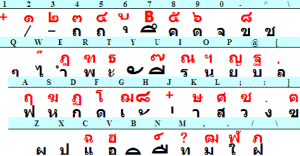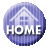Japanese

## Type Thai using VBA

Hear you can insert Thai characters in a Word document as you feel like keyboard touch. As VBA code used here is quite simple, you can readily alter to another language, for instance العربية Arabic and മലയാളം Malayalam languages.

### Argorithm and Alteration

Fig.1 Thai keyboardTable 1. Unicode and Characters
 char Dec Hex keyboard 1 ก 3585 0E01 d 2 ข 3586 0E02 - 3 ฃ 3587 0E03 ] 4 ค 3588 0E04 8 5 ฅ 3589 0E05 } 6 ฆ 3590 0E06 S 7 ง 3591 0E07 : 8 จ 3592 0E08 0 9 ฉ 3593 0E09 C 10 ช 3594 0E0A ^ 11 ซ 3595 0E0B + 12 ฌ 3596 0E0C G 13 ญ 3597 0E0D P 14 ฎ 3598 0E0E E 15 ฏ 3599 0E0F D 16 ฐ 3600 0E10 ` 17 ฑ 3601 0E11 R 18 ฒ 3602 0E12 < 19 ณ 3603 0E13 I 20 ด 3604 0E14 f 21 ต 3605 0E15 9 22 ถ 3606 0E16 5 23 ท 3607 0E17 m 24 ธ 3608 0E18 T 25 น 3609 0E19 o 26 บ 3610 0E1A @ 27 ป 3611 0E1B x 28 ผ 3612 0E1C z 29 ฝ 3613 0E1D / 30 พ 3614 0E1E r 31 ฟ 3615 0E1F a 32 ภ 3616 0E20 4 33 ม 3617 0E21 , 34 ย 3618 0E22 p 35 ร 3619 0E23 i 36 ฤ 3620 0E24 A 37 ล 3621 0E25 [ 38 ฦ 3622 0E26 ? 39 ว 3623 0E27 ; 40 ศ 3624 0E28 L 41 ษ 3625 0E29 K 42 ส 3626 0E2A l 43 ห 3627 0E2B s 44 ฬ 3628 0E2C > 45 อ 3629 0E2D v 46 ฮ 3630 0E2E V 47 ฯ 3631 0E2F O 48 ะ 3632 0E30 t 49 ั 3633 0E31 y 50 า 3634 0E32 k

… in-between has cut　…
 char Dec Hex keyboard 81 ๑ 3665 0E51 " 82 ๒ 3666 0E52 # 83 ๓ 3667 0E53 \$ 84 ๔ 3668 0E54 % 85 ๕ 3669 0E55 ( 86 ๖ 3670 0E56 ) 87 ๗ 3671 0E57 _ 88 ๘ 3672 0E58 = 89 ๙ 3673 0E59 ~ 90 ๚ 3674 0E5A 91 ๛ 3675 0E5B

Fig.1 shows an example of Thai keyboard. Character number, Thai character, and corresponding Unicode number are listed in Table 1. The fifth column in the table is location of keyboard character.

Algorithm is quite simple, i.e. if you type "d", then ChrW(3585) ( ก : Ko-Kai 1st Thai character ) turns out at the selected position.

In practical use, phrases are touch-typed in "InputBox" and push Enter key.

−　to alter to another language　−

Simply change variables "a" and "w", that's everything OK.

−　for example　−

If let "a" and "w" variables as follow, you can type Malayalam language.

```　　a = 3330
w = " x =  D E F R G T ~   Z S W   A Q k K i I U" & _
" ; + p P { : * @ ` C l L o O v  h H y Y c / j J" & _
" n N B b M < m u     e f r g t ^   z s w   a q d" & _
"                   |      0 1 2 3 4 5 6 7 8 9         "
```

and if you change as follow

```　 a = 1548
w = " K               P    ?  x N H c Y  h f m j e @ p o [  v . s a w q : / u" & _
" y       t r ; g l  i , n d W R A Q E S                X   "
```

you can type Arabic characters. Characters may be inserted left wise.

```　 a = 1025
W = " ?               F < D U L T + P B Q R K V Y J G H C N E A ` W X I O" & _
" { S M * > Z f M d u l t ; p b q r k v y j g h c n e a @ w x i o [ s m : . z _ "
```

becomes Russian language.

==== Guide to prepare Original one ====

−　Create original Table　−

Using Excel application, column 3 in Table 1. is newly prepared utilizing autofill technique. and column 4 are typed as 『 = dec2hex(c2) 』.

−　Create kAr()　−

As it needs rather high technique to create variable "w", following description may be simpler and better.

```kAr(1)="d": kAr(2)="-": kAr(3)="]": kAr(4)="8": kAr(5)="}": ……
```

In this case, the first line in VBA code must be changed as 『 Dim kAr(##) 』. and 『 kAr = Split(w) 』 is needed to delete. ( ## is an Unicode range: ## = 91 for Thai as shown in Table 1.)

### Digression

Keyboard character may differ depending on computer machine, so if needed, Array variable "kAr()" must be changed.

『 .InsertAfter vbCr: .Start = .End - 1: .Delete 』 is only a dummy, in the absence of this description, characters do not appear unless closing InputBox.

If you use Excel application, lines 16 to 18 must be changed as follow.

ActiveCell.Value = s: ActiveCell.Range("a2").Activate

```
Sub typethai()
Dim kAr                  '== Word type Thai Ver. 3.2　=====                                 ' 1
w = " d - ] 8 } S : 0 C ^ + G P E D ` R < I f 9 5 m T o @ x z / r a 4 , p i A [ ? ; L" & _
" K l s > v V O t y k e b u 7 n 6 &      ' g c F . w  q H j h U J N    Q " & Chr(34) & _
" # \$ % ( ) _ = ~ "                                                                 '4
kAr = Split(w)                                                                              '5
a = 3585: x = 100: y = 100: w = "The longest name of city": kAr(0) = "di6:gmrO"            '6
myLoop:                                                                                      '7
s = InputBox(kAr(0) & " will turns out " & w & _
vbCr & "Cansel for stop", "Type In", kAr(0), x, y)                                '9
w = "same as befor": kAr(0) = s:   If s = "" Then End                                 '10

For i = 1 To UBound(kAr)                                                               '13
s = Replace(s, kAr(i), ChrW(a + i - 1))                                            '14
Next                                                                                   '15
With Selection                                                                           '16
.InsertAfter Text:=s: .InsertAfter vbCr: .Start = .End - 1: .Delete                    '17
End With                                                                                 '18
GoTo myLoop                                                                               '19
End Sub

```

### このサイトで扱った項目